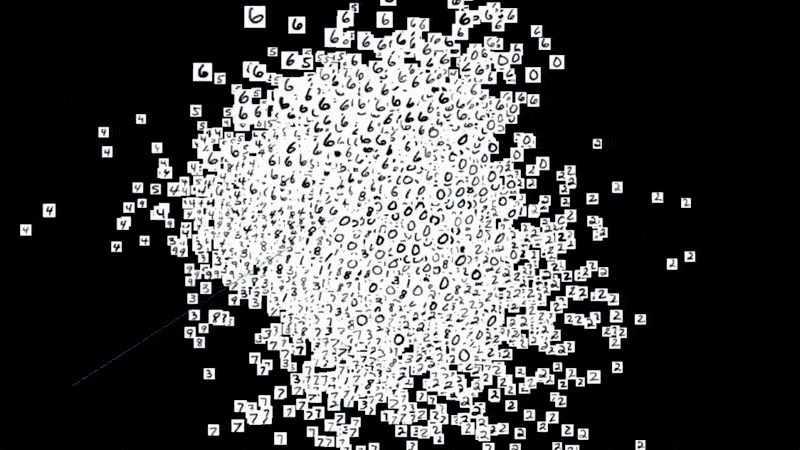# Projecting Your Data

## OverviewAn embedding is a map from input data to points in Euclidean space. In most machine learning applications, the model will transform high-dimensional vectors into a relatively low-dimensional space to make other processes. For example, in the case of image classification, usually, the model needs to transform the input image into a high-dimensional vector known as embeddings after a series of operations and identify the category of the input image based on the value of the embedding output from the model. At the same time, the embeddings from the same category should be close to each other in the high-dimensional space. In other words, the Euclidean distance from the same category should be minimized. And Researchers often need to explore the properties of a specific embedding to understand the behavior of their model. For these users, gaining an understanding of embedding geometry is a key step in interpreting a machine learning model.

One of the most approachable ways to analyze embeddings is to represent them visually. However, since embeddings usually exist as high-dimensional vectors, it is necessary to use dimensionality reduction to project the embeddings into a 2D or 3D space that humans can easily understand and analyze.

In this assignment, students need to build a classification model using the MNIST dataset and build an interactive embedding projector using tensorboard. Finally, students are required to interpret the behavior of the model based on different dimensionality reduction algorithms.

Instructors are allowed to modify the sample program based on their needs, including changing the dataset or the model architecture, etc.

 Summary Students build a Convolutional Neural Network (CNN) based on the MNIST dataset and build an interactive visualizer of high-dimensional data from the model's outputs. While doing so, students learn to investigate the properties of a specific embedding and the behavior of their model. Topics Convolutional Neural Networks, image classification, dimensionality reduction, data visualization. Audience Students who have learned introductory machine learning that covers the concepts of training data, test data, modeling, classification, prediction accuracy, and basic ideas about artificial neural networks. Difficulty This is an intermediate to advanced assignment, and denpends on student's comfort in programming, understanding . This assignment is designed for student to complete in a week with an instructor or teaching assistens present to provide help. Strengths This assignment covers the process from pre-processing the data to building the model and analyzing the predicted results. By completing these assignments, students are able to learn the practical application development process. The interactive embedding projector allows students to explore and analyze the relationship visually between the dataset and the model more intuitively. Weaknesses Descriptions and explanations are platform-specific (Pytorch). Preliminary knowledge of deep learning, especially image classification, is essential. Dependencies Learners are expected to have experience in building a machine learning model for classification, preferably for image classification. Intermediate to advanced levels of knowledge in machine learning, including artificial neural networks, convolutional neural networks, loss function, and embedding are required or should be taught in the adopting course. Mathematical competences in geometry, dimension reduction, and linear transformation are highly recommended. For the programming exercises, knowledge of Python programming, scikit‐learn, pytorch, and Jupyter notebooks are needed. Variants Instructors are able to modify the dataset, neural network, and different dimensionality reduction algorithms used to analyze the embeddings from the model to demonstrate different ways for exploring the data.

## Code Sample

We provide two types of sample code that can be executed in different environments for the instructor and the students to refer.

### Local Machine

Ensure that the packages listed in requirements.txt are installed, and the 6006 port can be exposed to start the TensorBoard service to watch the projecting results.